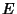## Completely Regular Graph

A Polyhedral Graph is completely regular if the Dual Graph is also Regular. There are only five types. Letbe the number of Edges at each node,the number of Edges at each node of the Dual Graph,the number of Vertices,the number of Edges, andthe number of faces in the Platonic Solid corresponding to the given graph. The following table summarizes the completely regular graphs.

 TypeTetrahedral 3 3 4 6 4 Cubical 3 4 8 12 6 Dodecahedral 3 5 20 39 12 Octahedral 4 3 6 12 8 Icosahedral 5 3 12 30 20